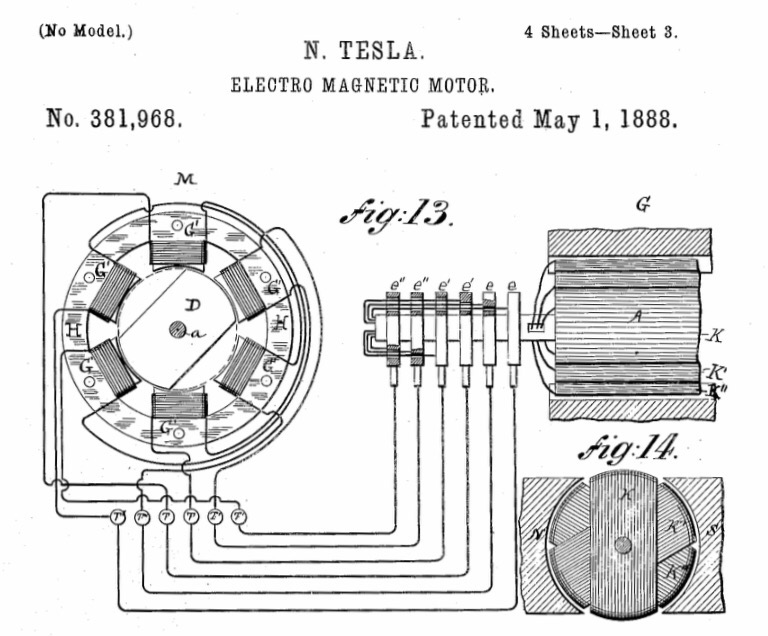## Watts so confusing?Most motors are designed to set amount of work, usually rated in either watts or horsepower, which is  746 watts per HP.

Watts law states that Watts = Volts x Amps. If a particular motor needs to do 1 horsepower of work at 120 Volts it will draw about 6.22 amps. And yes in an inductive load like a motor it’s not quite as simple as VxA=P but we are keeping it simple here.

A motor designed to do the same amount of work (1HP) at 240v will draw half the Amps (3.11).

This does not make the second motor “more efficient” because the power company charges by the Kilowatt NOT by the amp.

) If you take a load that is designed for a particular voltage and you DROP the voltage it will also decrease the wattage according to Watts law (Watts = Volts x Amps) as well as decrease the amperage according to Ohm’s law (so long as the resistance remains the same).

Let’s say you take a 5KW heat strip that is rated as 5Kw at 240v and you instead connect it to 120v.

It would then only produce 1.25 kw and draw 1/4 the amps, this is because while we may call it a “5 Kilowatt heater” it is actually just a fixed resistor designed to do 5 kilowatts per hour of work in the form of heat at 240 Volts. Cut the Volts in half you also cut the amps in half and you decrease the amount of work done down to 1/4 because Watts = Volts x Amps.

— Bryan

This site uses Akismet to reduce spam. Learn how your comment data is processed.

Scroll to top
Translate »

### Daily Tech Tip

Get the (near) daily Tech Tip email right in your inbox!